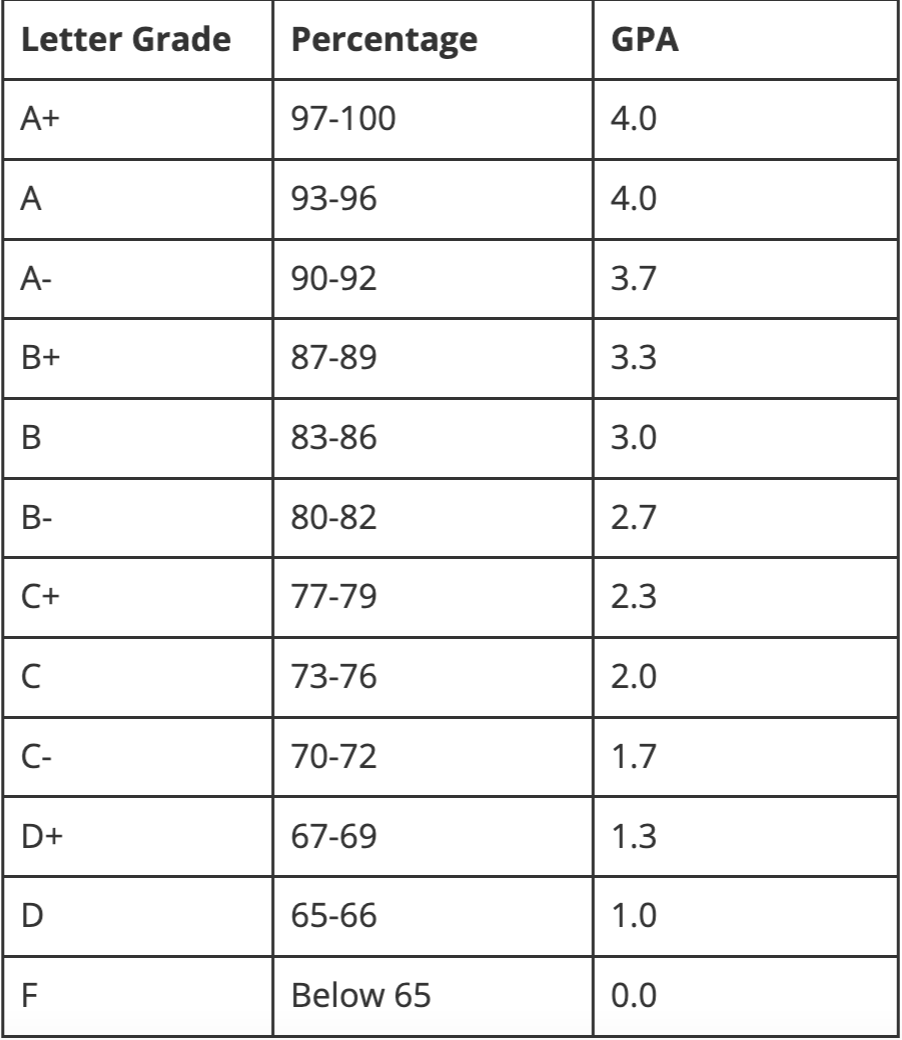top of page### GPA features

It does NOT account for the difficulty of coursework. What unweighted GPA consider is the grade students get from any class.

### GPA scale

0-4.0

The maximum GPA you can acquire is 4.0 if it is an unweighted GPA.

### GPA calculation

The GPA calculation can be easily done. If students know their grades and credits of all classes, they can get their unweighted GPA.

### GPA interpretation (+examples)

The chart in the right is our school GPA conversion chart.## Basic Information

### ​GPA features

It accounts for the difficulty of students; coursework. And the highest GPA students can receive vary according to the academic level of their classes.
Mostly, the classes are divided into 3 types: AP classes, honor classes, and regular classes.

### GPA scale

0-5.0

The maximum GPA you can acquire is 5.0 if it is a weighted GPA.

### GPA calculation

The GPA calculation may be tricky due to a variety of courses at different academic levels. Each grade has to be considered in conjunction with class level

### GPA interpretation (+examples)

1. The A in a regular-level class would still be a 4.0

2. The A in an honors class would be a 4.5

3. The two Bs in AP classes would each be 4.0s

4. The B in the honors class would be a 3.5

bottom of page